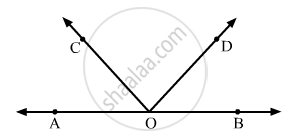# In the Given Figure, Aob Is a Straight Line. If ∠Aoc + ∠Bod = 85°, Then ∠Cod = - Mathematics

MCQ

In the given figure, AOB is a straight line. If ∠AOC + ∠BOD = 85°, then ∠COD =• 85°

• 90°

• 95°

• 100°

#### Solution

It is given that AOC is a straight line.Also, ∠AOC, ∠BOC, and ∠COD form a linear pair.

Therefore, their sum must be supplementary.

That is

∠AOC + ∠BOD+ ∠COD= 180°  ....(1)

It is given that

$\angle AOC + \angle BOD = 85°$      ......(2)
On substituting the value of (2) in (1) we get,

$\angle COD + 85^\circ = 180^\circ$

$\Rightarrow \angle OD = 180^\circ - 85^\circ$

$\Rightarrow \angle COD = 95^\circ$

Concept: Concept of Parallel Lines
Is there an error in this question or solution?

#### APPEARS IN

RD Sharma Mathematics for Class 9
Chapter 10 Lines and Angles
Q 6 | Page 51